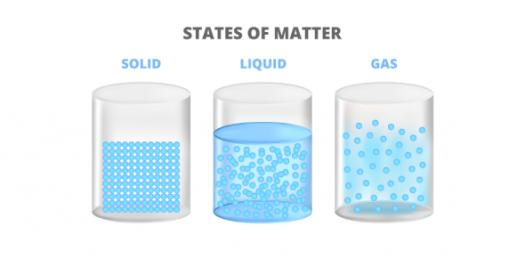# Mix And Flow Of Matter

9 Questions | Attempts: 3483SettingsA grade 8 level Quiz about mix and flow of matter, WHIMS, Cells and Systems, Light and Optical System, mechanical System, and Fresh and Saltwater Systems.

• 1.
Pressure is measured in ___ Work is measured in____ Force is measured in _____
• A.

Newtons,Pascales,Joules

• B.

Pascals,Joules,Newtons

• C.

Joules,Pascals,Newtons

• D.

Ml,g,ml squared.

• E.

None of the above

• 2.
When air is released in a submarine it is able to dive.This process enable the sumbarine to
• A.

Decrease the viscosity

• B.

Decrease its pressure

• C.

Increase its buoyancy

• D.

Increase its density

• E.

Decrease its pressure

• 3.
Condensation is The state in which a _______ goes to a _____
• A.

Gas to Solid

• B.

Liquid to Solid

• C.

Liquid to Gas

• D.

Solid to Gas

• E.

Gas to Liquid

• 4.
When you measure how thick or thin something is your measure how _____it is,
• A.

Viscouse

• B.

Dense

• C.

Buoyant

• D.

None of the above

• 5.
What is a solution?
• A.

When a solute dissolves in a solvent

• B.

When a colloid dissolves in a solvent

• C.

When a Solvent dissolves a solute

• D.

When a colloid dissolves in a solute

• E.

All the above

• 6.
Fluid A has a flow rate of 9.2ml, per second, Fluid B has a flow rate of 10.5ml, per second. Compared to Fluid A, fluid B is...
• A.

The same

• B.

More Dense

• C.

More viscouse

• D.

Less Dense

• E.

Less Viscouse

• 7.
All of the following are ways to increase Rate of Dissolving, exept...
• A.

Aggitation

• B.

Increase tempreture

• C.

Saturation

• D.

Decrease tempreture

• E.

Surface area

• 8.
If it takes 60 seconds for 12 ml of dirty oil to flow out of an engie, the flow rate is....
• A.

0.2

• B.

20

• C.

5

• D.

0.5

• E.

720

• 9.
The reason rocks feel heavier in water than in air is beacuse of...
• A.

Buoyant force of rock

• B.

Buoyant force of water

• C.

Densirty of water

• D.

Mass of rock and water together

• E.

None of the above

## Related TopicsBack to top
×

Wait!
Here's an interesting quiz for you.